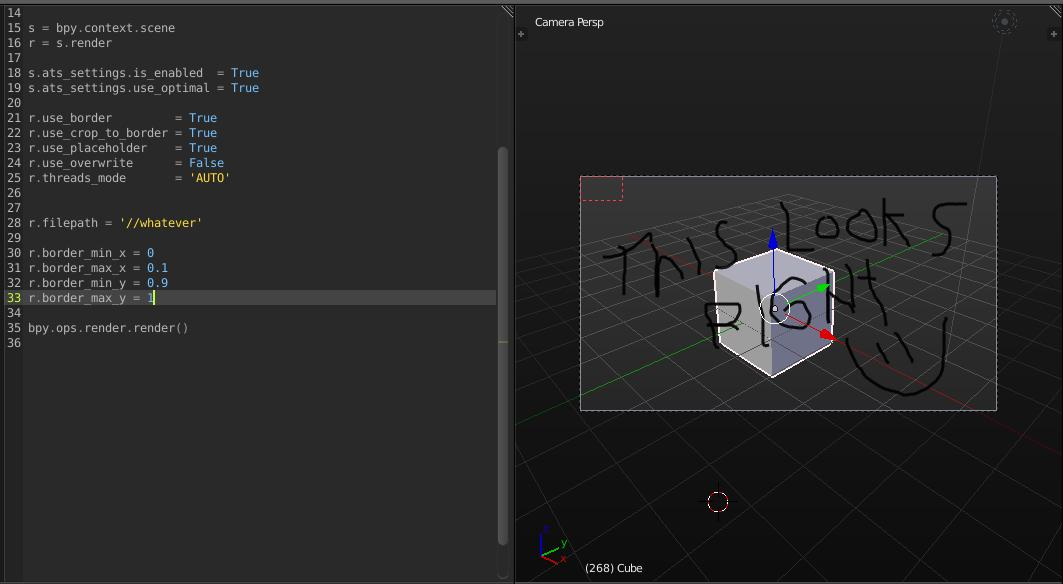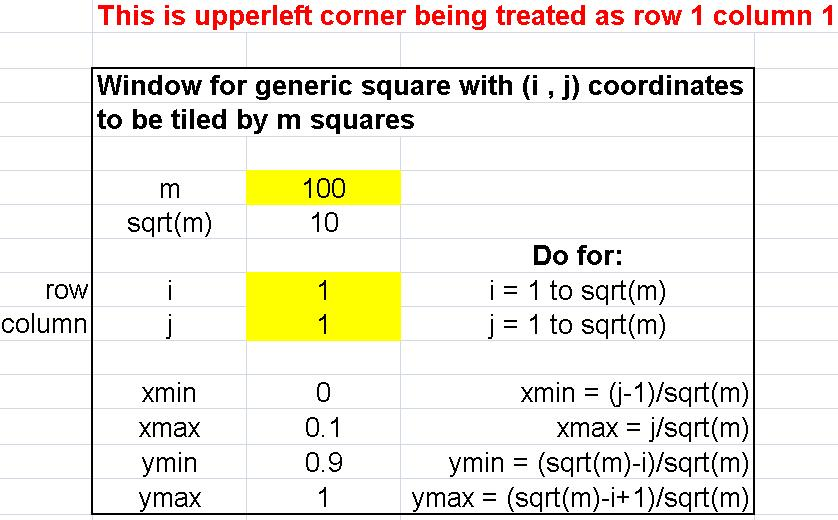If you find any of this useful, please consider donating via PayPal to help keep this site going.# Tiling a Square

6/12/16

Say you have a large image that you need rendered. It might be advantageous to you to split up that image and have each "tile" rendered on a different machine. Afterwards, you'd just have to stitch the parts back together. I recently helped my brother with the math for this.

Here is an example of a large image with one tile:I simpilifed the problem by assuming that the original image is a large square. We will be tiling this square by using m squares.

One interesting twist with this problem, is that the Blender program needs the "window" for each tile. So it isn't a matter of converting coordinates to new coordinates, but converting (i,j), where i is rows and j is columns, to xmin, xmax, ymin, and ymax, where these x and y min's and max's define a tile's window.

I recognized that if we have a square, and we're using m squares to tile, then m needs to be a square number. For example, 4, 16, 25, 36, etc. For example, if we use m = 100 squares, then we'll have a 10x10 grid that will tile the original square. Note that sqrt(100) = 10.

Therefore, to calculate the windows, we will go from i = 1 to sqrt(m) and j = 1 to sqrt(m) and calculate

• xmin = (j-1)/sqrt(m)
• xmax = j/sqrt(m)
• ymin = (i-1)/sqrt(m)
• ymax = i/sqrt(m)Another requirement, however, was that the Blender program needs the upperleft corner of the square treated as row 1 column 1. The standard math way, however, is treating the lowerleft corner as row 1 column 1, so I needed to change how the window was calculated.

Therefore, to calculate the windows, we will go from i = 1 to sqrt(m) and j = 1 to sqrt(m) and now calculate

• xmin = (j-1)/sqrt(m)
• xmax = j/sqrt(m)
• ymin = (sqrt(m)-i)/sqrt(m)
• ymax = (sqrt(m)-i+1)/sqrt(m)This was an interesting application of some basic math and I enjoyed it very much. Thank you for reading this.

If you enjoyed any of my content, please consider supporting it in a variety of ways: